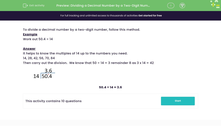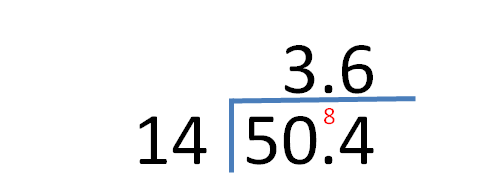# Divide a Decimal by a Two-Digit Number

In this worksheet, students will review and practise dividing decimal numbers by two-digit numbers.Key stage:  KS 3

Curriculum topic:   Number

Curriculum subtopic:   Use Four Operations for All Numbers

Difficulty level:#### Worksheet Overview

To divide a decimal number by a two-digit number, follow this method.

Example

Work out 50.4 ÷ 14

It helps to know the multiples of 14 up to the numbers you need:

14, 28, 42, 56, 70, 84

Then carry out the division.

We know that 50 ÷ 14 = 3 remainder 8 as 3 x 14 = 42

This gives us 84 ÷ 14 = 6 with no remainder.

We mustn't forget to line up the decimal point in the answer with its position in the calculation.50.4 ÷ 14 = 3.6

Shall we have a go at some questions now?

### What is EdPlace?

We're your National Curriculum aligned online education content provider helping each child succeed in English, maths and science from year 1 to GCSE. With an EdPlace account you’ll be able to track and measure progress, helping each child achieve their best. We build confidence and attainment by personalising each child’s learning at a level that suits them.

Get started